# NASH: Neural network architecture search based on rich network morphism and hill climbing algorithms | ICLR 2018

Posted Jun 15, 20205 min read

The paper proposes the NASH method for neural network structure search. The core idea is similar to the previous EAS method. The network morphism is used to generate a series of complex subnets with consistent effects and inherited weights. The network morphism in this paper is more abundant and only requires A simple hill climbing algorithm can complete the search, which takes 0.5 GPU day

Source:Xiaofei's algorithm engineering notes

Paper:Simple And Efficient Architecture Search for Convolutional Neural Networks# Introduction

The goal of the paper is to greatly reduce the amount of calculations for network search and maintain high performance of the results. The core idea is similar to the EAS algorithm, and the main contributions are as follows:

• Provide a baseline method, randomly construct the network and train with SGDR, and can achieve an error rate of 6%-7%on CIFAR-10, which is higher than most NAS methods.
• Expanded the research of EAS on network morphisms, which can provide popular network construction blocks, such as skip connection and BN.
• A neural network structure search NASH based on a hill climbing algorithm is proposed. This method iteratively performs network search. In each iteration, a series of network morphisms are used to obtain multiple new networks for the current network, and then cosine annealing is used for rapid optimization. Get a new network with better performance. On CIFAR-10, NASH only needs a single card for 12 hours to achieve baseline accuracy.

# Network Morphism

$\mathcal{N}(\mathcal{X})$is a series of networks on $\mathcal{X}\in \mathbb{R}^n$. The network morphism is Mapping $M:\mathcal{N}(\mathcal{X}) \times \mathbb{R}^k \to \mathcal{N}(\mathcal{X}) \times \ \mathbb{R}^j$, from the network with the parameter $w\in \mathbb{R}^k$$f^w \in \mathcal{N}(\mathcal{X})Convert to a network with the parameter \tilde{w} \in \mathbb{R}^j$$g^\tilde{w} \in \mathcal{N}(\mathcal{X})$, and satisfy Formula 1, that is, the output of the network remains unchanged for the same input.The following gives examples of network morphisms of several standard network structures:

### Network morphism Type I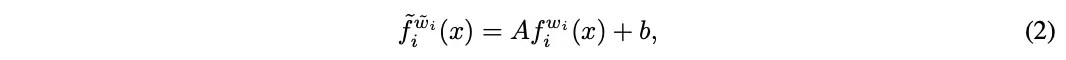Replace $f^w$with formula 2, $\tilde{w}=(w_i, C, d)$, in order to satisfy formula 1, set $A=1$and $b=0$, Can be used to add fully connected layers.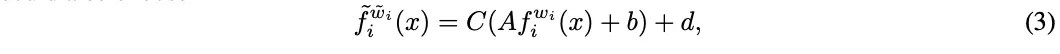Another complicated strategy is formula 3, $\tilde{w}=(w_i, C, d)$, set $C=A^{-1}$and $d=-Cb$, It can be used to express the BN layer, where $A$and $b$represent the statistical structure, and $C$and $d$are the learnable $\gamma$and $\beta$.

### Network morphism Type II

Assuming that $f_i^{w_i}$can be represented by any function $h$, ie $f_i^{w_i}=Ah^{w_h}(x)+b$Then you can combine $f^w$, $w_i =(w_h, A, b)$with any function $\tilde{h}^{w_{\tilde{h}}}(x)$Replace with $\tilde{f}^{\tilde{w}_i}$according to formula 4, $\tilde{w}=(w_i, w_{\tilde{h} }, \tilde{A})$, set $\tilde{A}=0$. This morphism can be expressed as two structures:

• To increase the layer width, imagine $h(x)$as the layer to be widened. Setting $\tilde{h}=h$can increase the layer width twice.
• The concatenation-type skip connection, assuming that $h(x)$itself is a series of layer operations $h(x)=h_n(x) \circ \cdots \circ h_0(x)$, set Set $\tilde{h}(x)=x$to achieve short-circuit connection.

### Network morphism Type IIIAny idempotent function $f_i^{w_i}$can be replaced by formula 5, initialize $\tilde{w}_i=w_i$, formula 5 is on the idempotent function without weight Also established, such as ReLU.

### Network morphism Type IV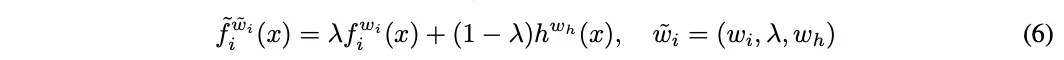Any layer $f_i^{w_i}$can be used with any function $h$to replace the formula 6, initialize $\lambda=1$, can be used to combine any function, especially non-linear function, can also be used For adding skip connection of additive type.
In addition, different network morphism combinations can also generate new morphisms. For example, the network structure of "Conv-BatchNorm-Relu" can be inserted behind the ReLU layer through formulas 2, 3 and 5.

# Architecture Search by Network Morphisms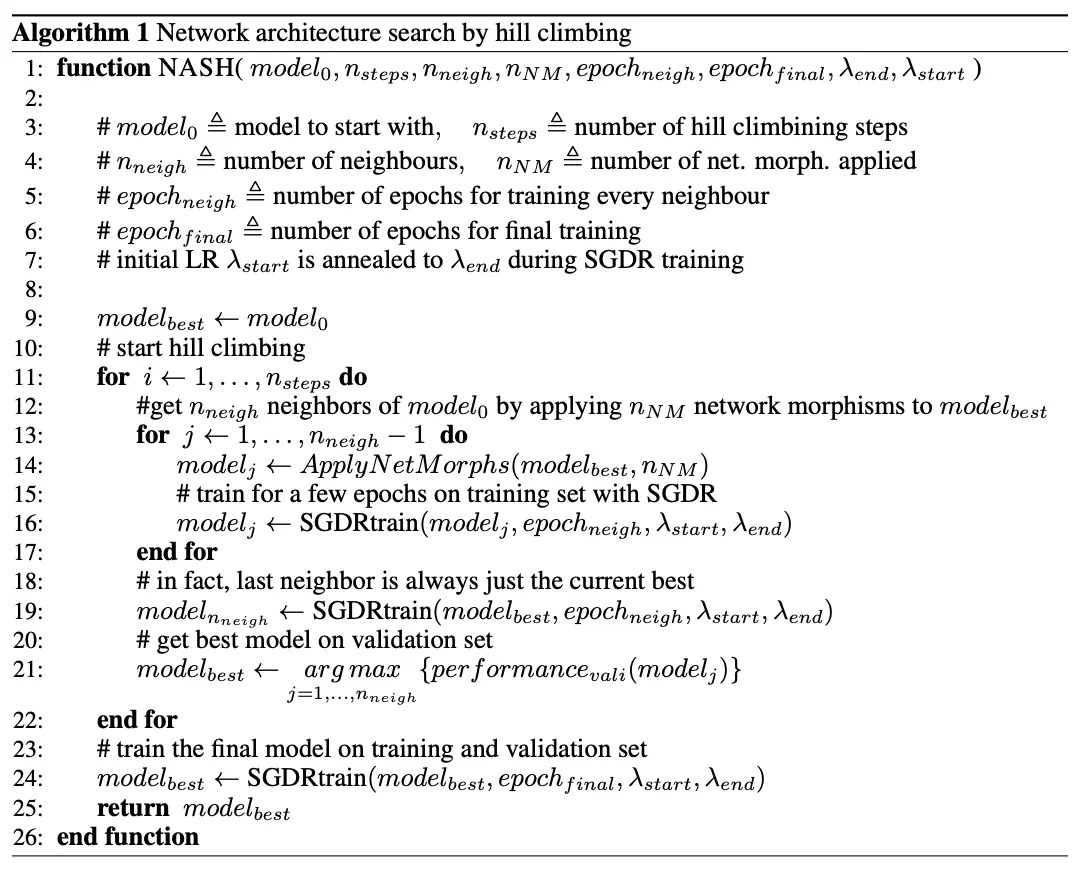The NASH method is based on a hill-climbing algorithm. It starts with a small network and performs network morphism on it to generate a larger subnet. Due to the constraint of Equation 1, the performance of the subnet is the same as the original network. Subsequent subnets are simply trained to see if they are Have better performance, and finally select a subnet with excellent performance for repeated operations.Figure 1 visualizes a step of the NASH method. The ApplyNetMorph(model, n) of Algorithm 1 contains n network morphism operations, each of which is a random one of the following methods:

• Deepen the network, such as adding the Conv-BatchNorm-Relu module, the insertion position and the size of the convolution kernel are random, and the number of channels is consistent with the most recent convolution operation.

• Widen the network, for example, use network morphism type II to widen the output channel, and the widening ratio is random.

• Add skup connection from layer $i$to layer $j$, use network morphism type II or IV, and the insertion position is randomly selected.

Due to the use of network morphism, the subnet inherits the weight of the original network and has the same performance. The advantage of the NASH method is that it can quickly evaluate the performance of the subnet. The paper uses a simple mountain climbing algorithm, of course, other optimization strategies can also be selected.

# Experiments

### Baslines### Retraining from Scratch### CIFAR-10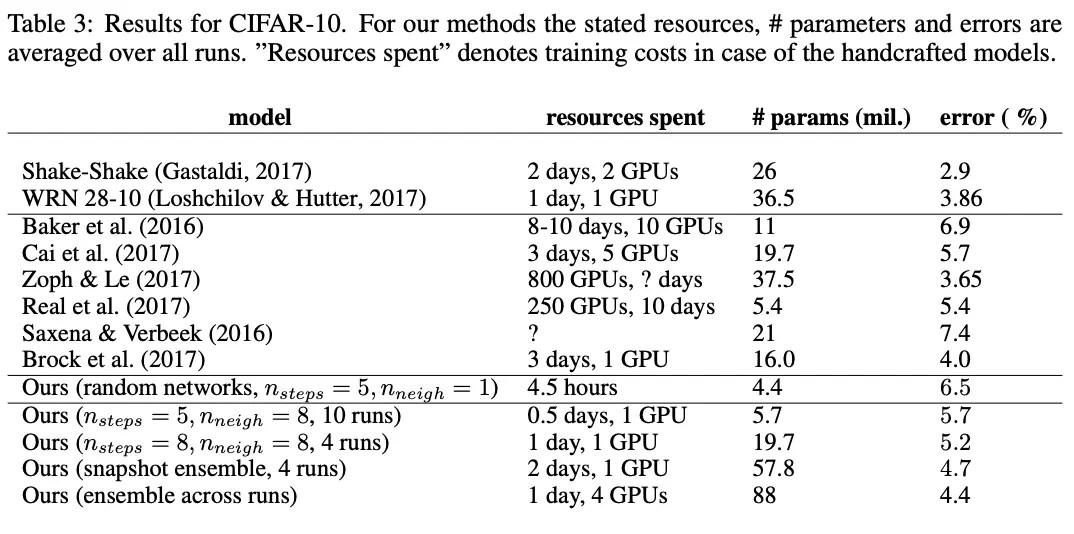### CIFAR-100# CONCLUSION

The paper proposes the NASH method for neural network structure search. The core idea is similar to the previous EAS method. The network morphism is used to generate a series of complex subnets with consistent effects and inherited weights. The network morphism in this paper is more abundant, and only requires simple The search can be completed with the aid of the mountain climbing algorithm, which takes 0.5 GPU day